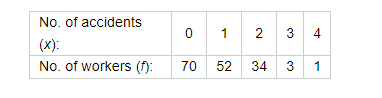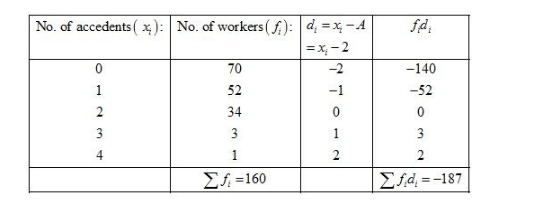# The following distribution gives the number of accidents

Question:

The following distribution gives the number of accidents met by 160 workers in a factory during a month.Find the average number of accidents per worker.

Solution:

Let the assume mean be $A=2$.We know that mean, $\bar{X}=A+\frac{1}{N} \sum_{i=1}^{n} f_{i} d_{i}$

Now, we have $N=\sum f_{i}=160, \sum f_{i} d_{i}=-187$ and $A=2$.

Putting the values in the above formula, we get

$\bar{X}=A+\frac{1}{N} \sum_{i=1}^{n} f_{i} d_{i}$

$=2+\frac{1}{160} \times(-187)$

$=2-\frac{187}{160}$

$=2-1.168$

$=0.83$

Hence, the average number of accidents per worker is 0.83.